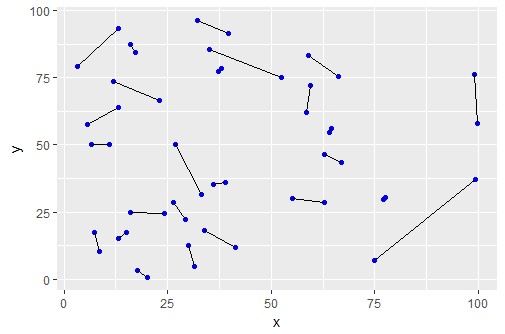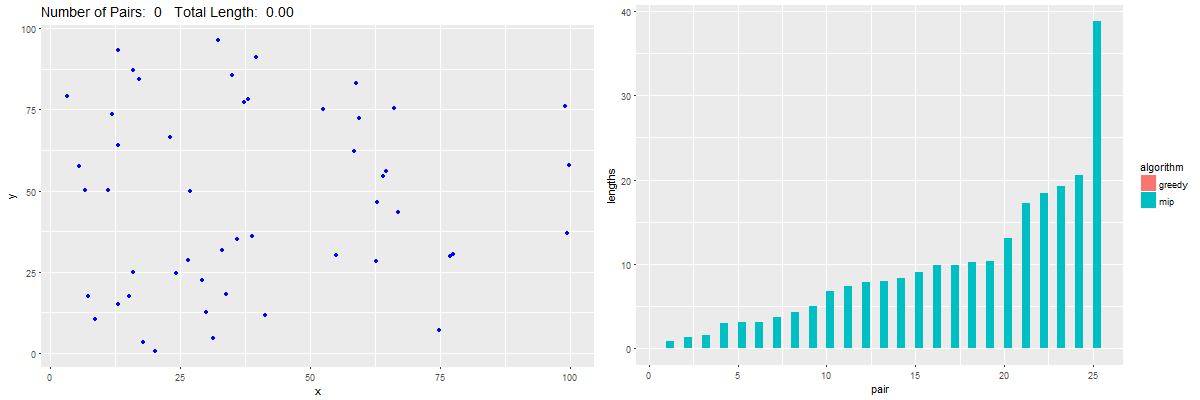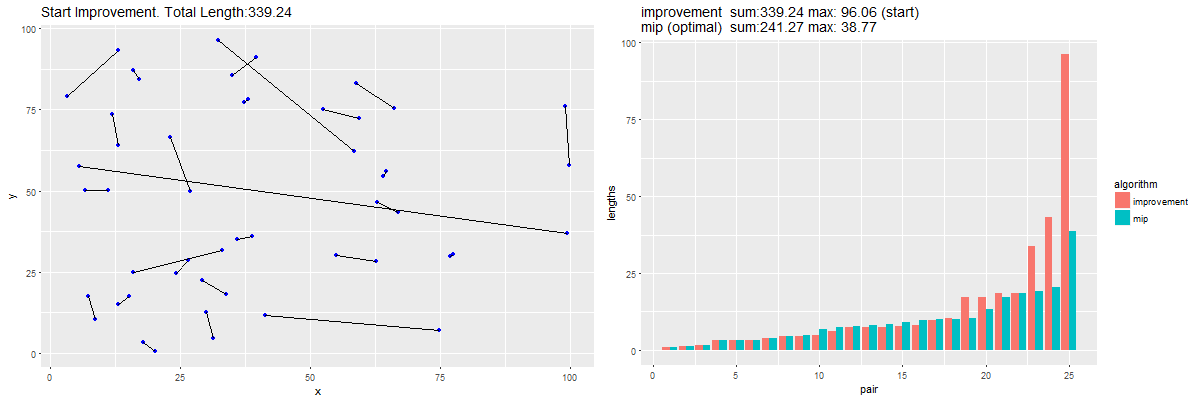## Saturday, January 11, 2020

### MIP vs greedy search

#### Problem

Assume we have $$N$$ points (with $$N$$ even). Find $$N/2$$ pairs of points, such that the sum of the lengths of the line segments based on these pairs, is minimized. This looks like an assignment problem where we do not know in advance the partition of the nodes in two equally sized sets. This problem is sometimes called a maximum matching on graphs

A picture is probably better than my arduous description:Optimal MIP solution: sum of the lengths is minimized

Let's try a MIP model.

#### MIP Formulation

The first thing to do is to calculate a distance matrix between points $$i$$ and $$j$$. As this matrix is symmetric, we only need to store the upper-triangular part (with $$i\lt j$$). That means we only need to store a little bit less than half the number of entries: $\mathit{ndist}=\frac{1}{2}N(N-1)$

If we use as variables $x_{i,j} = \begin{cases} 1 & \text{if nodes i and j are connected}\\ 0 & \text{otherwise}\end{cases}$ we need to think a bit about symmetry. If nodes 1 and 2 are connected, then we only want to see $$x_{1,2}=1$$ while ignoring $$x_{2,1}=1$$. So again, we only consider the variable $$x_{i,j}$$ when $$i \lt j$$. Again, this saves about half the number of variables we otherwise would use.

The model can look like:

MIP Model
\begin{align}\min& \sum_{i,j|i \lt j} \color{darkblue}d_{i,j} \color{darkred}x_{i,j}\\ & \sum_{j|j \gt i} \color{darkred}x_{i,j} + \sum_{j|j \lt i} \color{darkred}x_{j,i} = 1 && \forall i\\ & \color{darkred}x_{i,j} \in \{0,1\} \end{align}

The above picture is the optimal solution from this MIP model.

The constraint looks a bit strange. It basically says: every node $$i$$ is either a start- or an end-point of a single segment. Somewhat surprising, at first sight, is that this is enough to characterize our graph. Not many MIP models have just one single constraint block.

 Sidenote We can see in a picture how the constraint for $$i=4$$ is formed: The blue zone must contain exactly one 1. If we would pick $$j=7$$, i.e. $$x_{4,7}=1$$, the picture becomes:

It turns out this MIP model is fairly easy to solve. The example problem with $$N=50$$ points solves in a matter of seconds. For $$N=500$$ points, we get a large MIP with 124,750 binary variables and 500 constraints. Cplex solves this model quickly to optimality in 90 seconds on my laptop.

#### Greedy heuristic

An obvious greedy heuristic is as follows:

1. Find the pair with the shortest distance $\min_{i \lt j} d_{i,j}$ and record this segment.
2. Remove the two nodes that belong to this segment from the problem.
3. If there are still nodes left, go to step 1.

This heuristic is exceedingly simple to implement. Some results for our 50 point example:

MIP vs Greedy
PointsOptimal MIP ObjectiveGreedy Objective
50241.2667339.236

The greedy algorithm is really astonishingly bad. The animation below shows why: with a simple greedy algorithm we are doing very good initially, but pay the price in the end.Greedy algorithm in action

The picture on the left shows how my greedy algorithm selects shortest possible segment. The last few ones are really long. The right picture shows the lengths of the segment compared to the ones for the optimal MIP solution. The blue bars are formed by sorting the MIP solution. Until just before the end, things look honky-dory for the greedy algorithm. But the last few segments are really bad.

We can distinguish three areas:

• For the first, very small segments, the MIP solution and the greedy algorithm pick the same pairs. No difference.
• In the middle, the greedy algorithm is doing a little bit better: it picks shorter segments than the MIP solver.
• But at the end, the greedy algorithm totally collapses: it has to choose very long segments.

#### Improvement heuristic

We can follow the construction heuristic with an improvement heuristic. One simple one is just to consider two segments, and consider swapping them as follows:

If one of the alternatives has shorter segments, we perform the swap. We repeat this until no more improvement can be found.

Updating my plotting script leads to:An improvement heuristic

MIP vs Greedy+Improvement
AlgorithmObjective
MIP Model (optimal)241.27
Greedy339.24
Improvement269.66

This leads to much better results. The final sum of lengths is still a bit longer than optimal, but we actually have a shorter maximum length (again compared to optimal). The very long segments are gone. The difference compared to the optimal objective went from 40% (greedy algorithm) to 12% (improvement algorithm).

Notes:

• The improvement algorithm depends on the initial configuration. It may help to repeat it with a different (e.g. random) initial solution.
• This looks a bit like TSP nearest-neighbor and 2-opt heuristics.
• The MIP model only looks at minimizing the sum. We could alternatively minimizing the max, or using a combination of these two objectives. It is not unusual in practical models to combine min-sum with min-max.
• Needless to say the animations are not real time: lots of delays in order to be able to see what is happening.

This section was added later to this post.

#### References

1. Finding minimum total length of line segments to connect 2N points, https://stackoverflow.com/questions/59684772/finding-minimum-total-length-of-line-segments-to-connect-2n-points
2. Blossom Algorithm, https://en.wikipedia.org/wiki/Blossom_algorithm. Algorithm for matching problems.

1.I wonder how this greedy algorithm compares:

Define the potential as half the sum of each unconnected point's nearest neighbor distance. Then always pick the pair that minimizes the resulting sum of the potential and all line segment lengths.

(I've seen this algorithm or a very similar one for a similar problem before, but I don't remember where right now.)

2.For any crossing in an assignment you can always undo the crossing and thus reduce the total length. You keep doing this while there are crossings. Though not necessarily optimal, this will be a crossing-free final arrangement.

3.This is the maximum-weight independent set problem on the line graph.